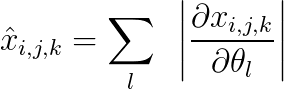Hi. I am looking for an efficient way of computing \hat{x} of dimensions (b x c x h x w) defined per sample as:.

where x is the output of the same dimensions generated by a model with parameters \theta, and

i,j: index the height and width of the 2D output feature map
k: indexes the channel dimension
l: indexes the parameters.

How do I accomplish this with x.backward()?

If I did x.backward(torch.ones_like(x)), I would compute the sum of the gradients in the above equation in the place of sum of their absolute values.

Is there an efficient way of doing this?

EDIT:
Brute-force way to do this would be to loop over each pixel in the output map and compute .backward(). But, that would be incredibly expensive. Is there an efficient way which avoids the loop?

Could you please have a look? Thank you. @smth @InnovArul @ptrblck

Please don’t tag people, as this might discourage others to answer in the topic.
We’ll have a look at all topics and try to help as best as we can1 Like